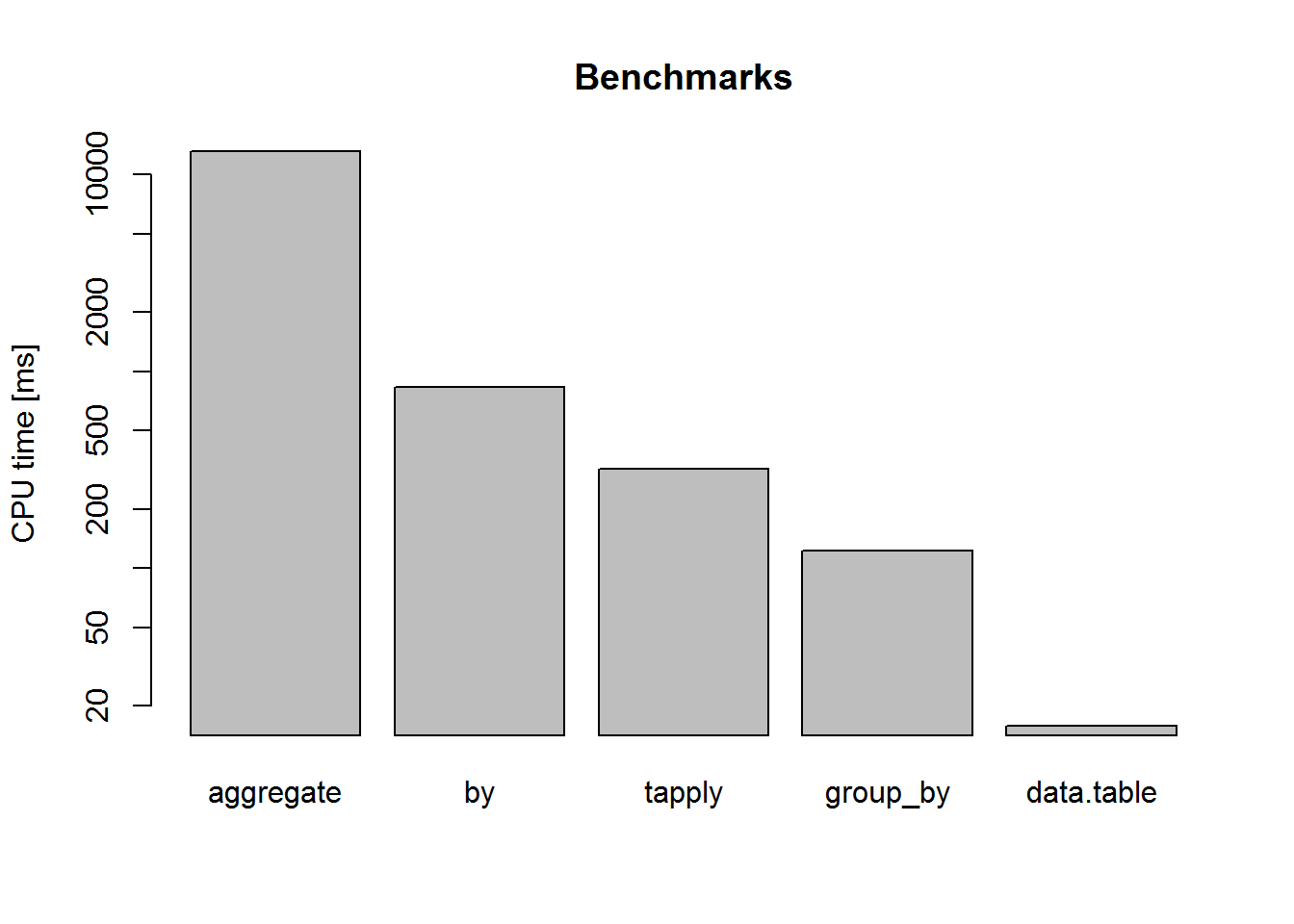# Aggregating data in R, benchmarks

#### Greatly varying processing times of aggregating data in R

While exploring the kaggle Rossmann competition (https://www.kaggle.com/c/rossmann-store-sales) with our class I stumbled upon this script: https://www.kaggle.com/shearerp/rossmann-store-sales/interactive-sales-visualization

It is a great introduction to the syntax that comes with the package dplyr.

The “model” proposed is simply the average sales conditioned on three variables: ‘Store’,‘DayOfWeek’,‘Promo’.

What I found remarkable is that the processing of this aggregation step is much faster than with the base function aggregate, which led me to benchmarking five different ways of conditional function computation:

1. base R
• aggregate
• by
• tapply
2. dplyr package: group_by_ %>% summarise
3. data.table package: [,by=]

We use the library microbenchmark to time the computation of average sales conditional on three factors in the Rossmann data set.

require(dplyr)
train = train[train$Sales>0,] dim(train) ##  844338 9 #define the variables we want to condition on preds=c('Store','DayOfWeek','Promo') library(microbenchmark) In summary, the differences are staggering: Benchmark = c(aggregate=mean(AggFormula[,2]), by=mean(BY[,2]), tapply=mean(TA[,2]), group_by=mean(GrpByPipes[,2]), data.table=mean(DTgroup[,2]))/10^6 round(Benchmark) ## aggregate by tapply group_by data.table ## 13169 835 319 123 16 barplot(Benchmark, log="y", ylab = "CPU time [ms]", main = "Benchmarks")The logarithmic plot hides the enormous speed differences: the data table grouping is 835 times faster than aggregate! GrpByPipes=microbenchmark( "mdl" = train %>% group_by_(.dots=preds) %>% summarise(PredSales=mean(Sales)) %>% ungroup(), times = 20) print(GrpByPipes) AggFormula =microbenchmark( "mdl2" = aggregate(Sales ~ Store + DayOfWeek+Promo, data=train,FUN=mean), times = 20) print(AggFormula) Does it help to bypass the formula overhead:? AggNoFormula =microbenchmark( "mdl3" = aggregate(train$Sales, list(train$Store, train$DayOfWeek, train$Promo),FUN=mean), times = 20) print(AggNoFormula) Hardly any difference. Are the alternatives to aggregate such as by, tapply faster? TA =microbenchmark( "mdl3b" = tapply(train$Sales, list(train$Store, train$DayOfWeek, train$Promo),FUN=mean), times = 20) BY =microbenchmark( "mdl3c" = by(train$Sales, list(train$Store, train$DayOfWeek, train$Promo),FUN=mean), times = 20) print(TA, eval=F) ## Unit: milliseconds ## expr min lq mean median uq max neval ## mdl3b 293.1418 305.0216 318.9019 310.9083 314.4047 415.5317 20 How does data.table compare to this? (slightly unfair to leave the indexing outside the benchmarking) library(data.table) DT= data.table(train) system.time(setkey(DT,Store,DayOfWeek,Promo)) DTgroup =microbenchmark( "mdl4" = DT[, mean(Sales), by="Store,DayOfWeek,Promo"], times = 20) print(DTgroup, eval=F) ## Unit: milliseconds ## expr min lq mean median uq max neval ## mdl4 15.68308 15.69807 15.77953 15.70956 15.84216 16.13794 20 And a linear model is not competitive at all when it comes to computing interactions only: train$Store=factor(train$Store) train$DayOfWeek=factor(train$DayOfWeek) train$Promo=factor(train\$Promo)
system.time(lm(Sales ~ Store:DayOfWeek:Promo, data=train))
print("Error: cannot allocate vector of size 98.2 Gb")
##  "Error: cannot allocate vector of size 98.2 Gb"